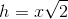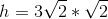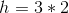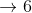# High School Math : How to find the length of the side of a 45/45/90 right isosceles triangle

## Example Questions

### Example Question #8 : 45/45/90 Right Isosceles Triangles

What is the area of a square that has a diagonal whose endpoints in the coordinate plane are located at (-8, 6) and (2, -4)?

100√2

200√2

100

50

50√2

100

Explanation: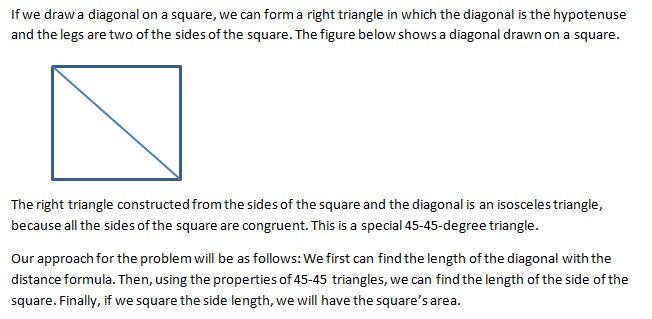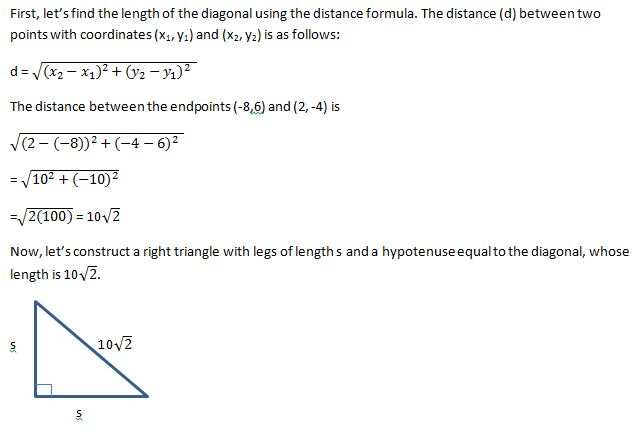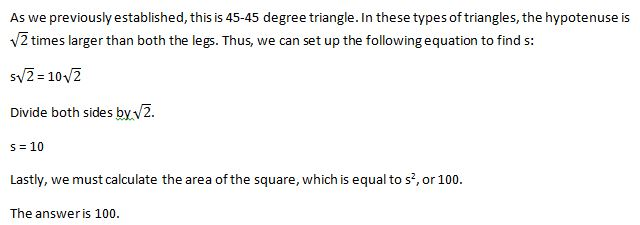### Example Question #21 : Isosceles Triangles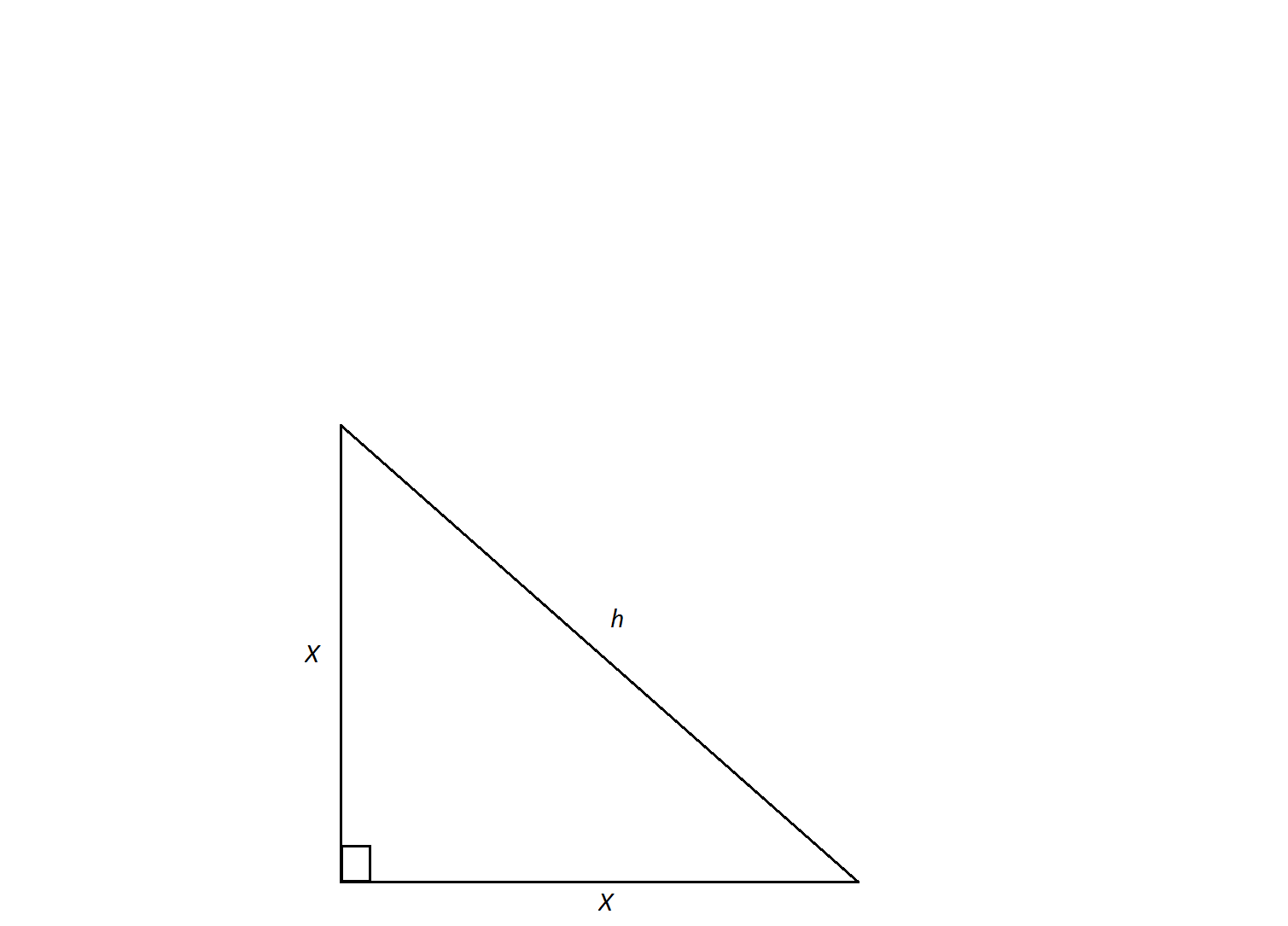An isosceles triangle has a hypotenuse of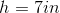. Find the length of its sides,.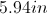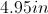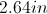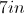Not enough information to solveExplanation:

An isosceles triangle is a special triangle due to the values of its angles. These triangles are referred to as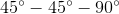triangles and their side lengths follow a specific pattern that states you can calculate the length of the legs of an isoceles triangle by dividing the length of the hypotenuse by the square root of 2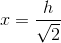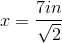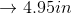### Example Question #31 : TrianglesThe measure of the sides of this isosceles right triangle are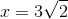. Find the measure of its hypotenuse,.Not enough information to solveExplanation:

An isosceles triangle is a special triangle due to the values of its angles.  These triangles are referred to astriangles and their side lenghts follow a specific pattern that states you can calculate the length of the hypotenuse of an isoceles triangle by multiplying the length of one of the legs by the square root of 2.